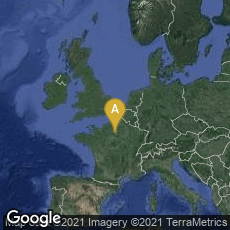A: Paris, Île-de-France, France

# François Viète's Classic of Mathematics and Typography

1579

In 1579 French lawyer, Conseil du Roi (privy councillor), and mathematician François Viète (Franciscus Vieta) published in Paris Canon mathematicus seu ad triangula. Cum adpendicibus.

Viète's numerous mathematical works were written during two brief periods of leisure from his career as a lawyer to the French courts of Henry III and Henry IV. His Canon mathematicus, the earliest of his published mathematical works, was the first of his studies on trigonometry.

"Here he gathered together the formulas for the solution of right and oblique plane triangles, including his own contribution, the law of tangents. . . . For spherical right triangles he gave the complete set of formulas needed to calculate any one part in terms of two other known parts, and the rule for remembering this collections of formulas, which we now call Napier's rule. He also contributed the law of cosines involving the angles of an oblique spherical triangle" (Kline, Mathematical Thought from Ancient to Modern Times  239-240).

In addition, Viète called for a reform in the expression of fractions, in which decimal fractions would replace the sexagesimal fractions then used in astronomy, physics and mathematics.

Viète's work consists of two parts: "Canon mathematicus," containing a table of trigonometric lines with some additional tables; and "Universalium inspectionum ad canonem mathematicum" (with separate title), giving the computational methods used in the construction of the canon and explaining the computation of plane and spherical triangles. Viète had originally planned to include two more parts devoted to astronomy, but these were never published.

Canon mathematicus was remarkably advanced typographically for its time. It is also very rare: privately printed in a small edition, its scarcity was compounded by Viète's displeasure over its many misprints, which caused him to withdraw from circulation all the copies he could recover.

Dibner, Heralds of Science, no. 105.  Hook & Norman, The Haskell F. Norman Library of Science and Medicine (1991) no. 2151.